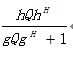# How to express this problem in CVX

Question:
first questiona,b,c,d are four different numbers,I don’t know how to express it in a constraint.

second question:Q is variable,h and g are row vectors,My expression is`h*Q*h/(1+g*Q*g)`,The error shows “Disciplined convex programming error:
Cannot perform the operation: {real affine} ./ {real affine}“

If `b` is not necessarily equal to `c`, this is just an elementwise inequality, which can be entered as [a b;c d] >= 0` or perhaps a small positive number such as 1e-5 instead of zero. CVX treats all inequalities as non-strict.
As for the 2nd question, you haven’t shown how the expression will be used. Look up `linear-fractional` at https://web.stanford.edu/~boyd/cvxbook/ .